# How To Size A Solar Panel For Your Battery

Posted on 02/23/2018 at 12:00 by Daniel Stieler PhdHow do you determine the power output required from a solar panel to recharge a battery based on the current load?

This is a great question and important when considering what solar panel to select for your system.

First, you need to determine the power required for your application. If you know the current load, say, for example, you have a device with an average current draw of 2A at 5V and operates for 2 hours per day.

To determine the number of watt-hours of power required to operate the device, use the following equation:

WattHours=OperatingVoltage*CurrentDraw*HoursofOperation

WattHours=5V*2A*2 Hours=20 WattHours

If this device is connected to a 12V battery and converted to 5V for the device's operation, then the efficiency of the 12V to 5V step-down converter will also need to be accounted for.

Converter efficiency can be measured by operating the system and measuring the voltage and current at the input and output of the converter. Since the device draws 2A at 5V, that is on the output side of the converter.

On the input side is the 12V battery.  Let’s say the input from the 12V battery measures to be 13V and 1A. To calculate the converter efficiency, use the following equation: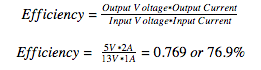(Most off-the-shelf, inexpensive converters will be around 65-70% efficient. Quality converters will be 90+ efficient)

Since the system has a converter, the converter efficiency must also be accounted for in the Watt-Hour calculation. This can be done in one of two ways.

The first way is using the original watt-hour equation and substituting the input voltage and current values.

Watt Hours=13V*1A*2 Hours=26 Watt Hours

The second way is to use the following equation: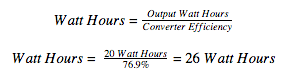In this example, 26 watt-hours would be the amount of power your solar panel would need to yield daily.

At this point, you need to determine solar radiation for the area the system will be used. There are multiple places online where you can get an estimate of this. For this example, let’s use NREL’s PVWatts calculator.

It will ask for the location, which in this example will be Ames, IA, home of PowerFilm. Then it will ask for several other parameters. To determine the solar radiation, the only necessary parameters to change are the installed tilt angle and the Azimuth angle.

For this example, it is assumed that the panel will be staked flat on the ground, so the Tilt will be 0, and the Azimuth will be 180.  The program will output a table that looks like the one below: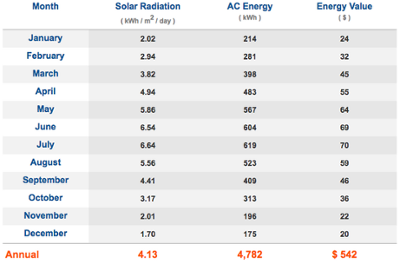To size a system to work continuously year round the solar will need to be sized for the worst-case month, which is December. In December, the average solar radiation is 1.7kWh/m2/day.  A solar panel’s performance is specified based on 1kW/m2, which means that a panel averages only 1.7 hours of sun per day. So, the minimum solar panel size can be determined using the following equation: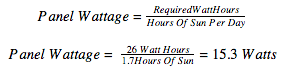This would be the minimum wattage and assumes that the panel will see the average solar radiation daily to maintain operation with no disruption. Realistically there will be sunny and overcast days, so a battery storage system will need to be specified to be large enough to provide power during extended periods of low solar radiation. Also, the panel will need to be larger to recharge the storage system during times of good solar radiation. The actual storage system and panel sizes will be based on application and tolerance to loss of power.

Using a standard 80 Amp-Hour lead acid battery, the system could operate off a full battery for 36.9 days.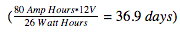To charge the battery from empty to full in 5 days with 1.7 hours of solar radiation, the required panel size would be 112 watts.For a charge current in amps greater than 1/100th the amp hour capacity of the battery, a charge controller will be needed. In this case, the charge current will be approximately 9.3A.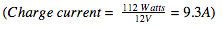This is > 1/100th the amp-hour charge capacity of an 80 Amp-Hour battery, so a charge controller will be needed to keep the battery from overcharging.

Adding a charge controller to the system will introduce additional parasitic loss. Charge controller efficiencies range from 60-90%. The panel size will need to be increased to account for this efficiency loss. If a 90% efficient charge controller is chosen, then the final panel size required will be given by the following equation: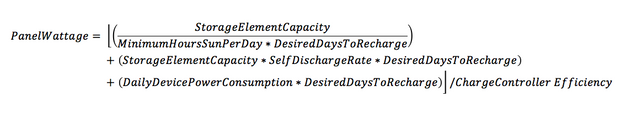So the next time you need to find the right size panel for your job, remember to determine your application’s power requirements and adjust for multiple factors: converters, any required controller, local irradiance, system tolerances and risk.

Thinking through your power solution critically and completely on the front end will save time and headaches down in the future.

Need some help designing a custom solar solution for your next project?

We would love to have a conversation.

We look forward to hearing from you and working through your power needs.